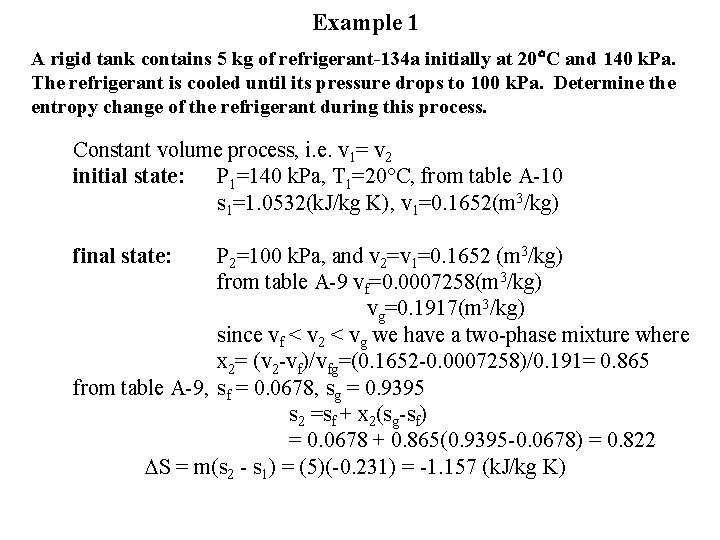Entropy of a Pure Substance Entropy is a

• Slides: 4Entropy of a Pure Substance • Entropy is a thermodynamic property, i. e entropy only depends on the state of the system. Example: given T & P, entropy, S, can be obtained from a thermodynamic table just like v, u, h. • If the state corresponds to a liquid/vapor mixture, entropy can be determined as: s = sf + xsfg, where x is the quality and sfg are saturated values listed in the saturation table. • For an incompressible liquid, the entropy can be approximated by the entropy of the saturated liquid at the given temperature since it is not a function of pressure: [email protected], P [email protected] P = const. T v = const s (k. J/kg)Example 1 A rigid tank contains 5 kg of refrigerant-134 a initially at 20 C and 140 k. Pa. The refrigerant is cooled until its pressure drops to 100 k. Pa. Determine the entropy change of the refrigerant during this process. Constant volume process, i. e. v 1= v 2 initial state: P 1=140 k. Pa, T 1=20 C, from table A-10 s 1=1. 0532(k. J/kg K), v 1=0. 1652(m 3/kg) final state: P 2=100 k. Pa, and v 2=v 1=0. 1652 (m 3/kg) from table A-9 vf=0. 0007258(m 3/kg) vg=0. 1917(m 3/kg) since vf < v 2 < vg we have a two-phase mixture where x 2= (v 2 -vf)/vfg=(0. 1652 -0. 0007258)/0. 191= 0. 865 from table A-9, sf = 0. 0678, sg = 0. 9395 s 2 =sf + x 2(sg-sf) = 0. 0678 + 0. 865(0. 9395 -0. 0678) = 0. 822 DS = m(s 2 - s 1) = (5)(-0. 231) = -1. 157 (k. J/kg K)Isentropic Process • Isentropic process: entropy is constant, hence Ds=0. A reversible, adiabatic process is always isentropic since there is no entropy generation due to irreversibilities (sgen=0) and no change of entropy due to heat transfer (ds= Q/T=0). • The reverse is not always true - an isentropic process is not necessary a reversible, adiabatic process. Why? • A Carnot cycle consists of two isentropic processes: reversible, adiabatic compression and expansion plus two isothermal processes. T isothermal Isentropic s=constant sExample 2 Steam enters an adiabatic turbine at 5 MPa and 450 C and leaves at a pressure of 1. 4 MPa. Determine the work output of the turbine per unit mass flowing through the turbine if we can assume the process is reversible and neglect all changes of KE and PE. T 5 MPa 1 2 1. 4 MPa s sg Wout = m(h 1 -h 2), Wout/m = h 1 -h 2 state 1: P 1=5 MPa, T 1=450 C, from table A-6 h 1=3316. 2(k. J/kg), s 1=6. 819(k. J/kg K) State 2: P 2=1. 4 MPa, s 2=s 1=6. 819>sg=6. 469 @ 1. 4 MPa(table A-5), state 2 is superheated: from table A-6 through interpolation h 2=2927. 2+[(3040. 4 -2927. 2)/(6. 9534 -6. 7467)](6. 819 -6. 7467)=2966. 8 (k. J/kg). Wout/m = h 1 -h 2 = 349. 4 (k. J/kg)